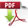×#### Thank you for registering.

One of our academic counsellors will contact you within 1 working day.

Click to Chat

1800-1023-196

+91-120-4616500

CART 0

• 0

MY CART (5)

Use Coupon: CART20 and get 20% off on all online Study Material

ITEM
DETAILS
MRP
DISCOUNT
FINAL PRICE
Total Price: Rs.

There are no items in this cart.
Continue ShoppingSolved Examples on Indefinite IntegralExample 1: If ∫ xex cos x dx = f(x) dx + c, then f(x) is equal to

∫ xe(1 + i)x / 1+i – ∫ e(1+i)x / 1 + i dx = xe(1+i)x / 1+ i – e(1+i)x / (1+i)2

e(1 + i)x[x(1 + i –1 / (1+i)2)]

ex (cos x + i sin x) [(x–1) + ix / 1 + i – 1]

ex/–2 [icos x – sin x][(x – 1) + ix]

I = ex/2 [(1 – x) sin x – x cos x] + c.

Example 2: Let x2 +1 ≠ nπ, n ∈ N, then

(a) ln |1/2 sec (x2 + 1) | + c

(b) ln | sec { ½ (x2 + 1) | + c

(c) 1/2 ln | sec (x2 + 1) | + c

(d) ln | sec (x2 + 1) | + c

Solution:  Let

I =

Put x2 + 1= t

x dx = ½ dt

Then I = ½ ∫ √ (2 sin t – sin 2t)/(2 sin t + sin 2t) dt

= ½ ∫ √ (1 - cos t)/(1 + cos t) dt

= ½ ∫ tan (t/2) dt

= ½ .2. ln | sec (t/2)| + c

= ln | sec {(x2+1)/2} + c

= ln | sec {1/2 (x2 + 1)} + c

Example 3: Let f(x) be a function such that f(0) = f’(0) = 0, f”(x)  = sec4x + 4, then the function is

(a) ln |(sin x)| + 1/3 tan3x + x

(b) 2/3 ln |(sec x)| + 1/6 tan2x + 2x2

(c) ln |cos x| + 1/6 cos2x – x2/5

(d) none of these

Solution: Since f”(x)  = sec4x + 4

So, f”(x)  = (1 + tan2x) sec2x + 4

Therefore, f’(x) = tan x + tan3x/3 + 4x + c

Since, f’(0) = 0

So, 0 = c

Then, f’(x) = tan x + + tan3x/3 + 4x

= tan x + 1/3 tan x (sec2x – 1) + 4x

f’(x) = 2/3 tan x + 1/3 tan x sec2x + 4x

So, f(x) = 2/3 ln | sec x| + tan2 x/6 + 2x2 + d

But, f(0) = 0

So, d = 0

Then, f(x) = 2/3 ln | sec x | + 1/6 tan 2x + 2x2.

Example 4: ∫ x-2/3 (1 + x1/2)-5/3 dx is equal to

(a) 3(1 + x-1/2)-1/3 + c

(b) 3(1 + x-1/2)-2/3 + c

(c) 3(1 + x1/2)-2/3 + c

(a) none of these

More than one

Example 5:  more than one

If

(a) A = 3/2

(a) B = 35/36

(a) C is indefinite

(a) A + B = -19/36

Example 6: Fill in the blanks: ∫ √ x + √(x2 + 1) dx is equal to

Example 7: True/false: The antiderivative of f(x) = ln(ln x) + (ln x)-2 whose graph passes through (e, e) is x ln(ln x) – x(ln x)-1.

Solution:Download IIT JEE Solved Examples of Indefinite Integral

To read more, Buy study materials of Indefinite integral comprising study notes, revision notes, video lectures, previous year solved questions etc. Also browse for more study materials on Mathematics here.### Course Features

• 731 Video Lectures
• Revision Notes
• Previous Year Papers
• Mind Map
• Study Planner
• NCERT Solutions
• Discussion Forum
• Test paper with Video Solution